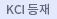#검색
다국어 입력

http://chineseinput.net/에서 pinyin(병음)방식으로 중국어를 변환할 수 있습니다.

변환된 중국어를 복사하여 사용하시면 됩니다.

예시)
• 中文 을 입력하시려면 zhongwen을 입력하시고 space를누르시면됩니다.
• 北京 을 입력하시려면 beijing을 입력하시고 space를 누르시면 됩니다.
닫기

검색결과 좁혀 보기

• 좁혀본 항목 보기순서

• 원문유무
• 음성지원유무
• 원문제공처
• 등재정보
• 학술지명
• 주제분류
• 발행연도
• 작성언어
• 저자

오늘 본 자료

• 오늘 본 자료가 없습니다.
더보기
• 무료
• 기관 내 무료
• 유료
•ERROR ESTIMATES FOR FULLY DISCRETE DISCONTINUOUS GALERKIN METHOD FOR NONLINEAR PARABOLIC EQUATIONS

In this paper, we develop discontinuousGalerkinmethods with penalty terms, namaly symmetric interior penalty Galerkin methods to solve nonlinear parabolic equations. By introducing an appropriate projection of u onto finite ele-ment spaces, we prove the optimal convergence of the fully discrete discontinuous Galerkin approximations in ℓ²(L²) normed space.

•A PRIORI L² ERROR ANALYSIS FOR AN EXPANDED MIXED FINITE ELEMENT METHOD FOR QUASILINEAR PSEUDO-PARABOLIC EQUATIONS

Based on an expanded mixed finite element method, we con- sider the semidiscrete approximations of the solution u of the quasilinear pseudo-parabolic equation defined on Ω ⊂ R d , 1 ≤ d ≤ 3. We construct the semidiscrete approximations of ∇u and a(u)∇u + b(u)∇u t as well as u and prove the existence of the semidiscrete approximations. And also we prove the optimal convergence of ∇u and a(u)∇u + b(u)∇u t as well as u in L² normed space.

•HIGHER ORDER DISCONTINUOUS GALERKIN FINITE ELEMENT METHODS FOR NONLINEAR PARABOLIC PROBLEMS

In this paper, we consider discontinuous Galerkin finite element methods withinterior penalty term to approximate the solution of nonlinear parabolic problems with mixedboundary conditions. We construct the finite element spaces of the piecewise polynomialson which we define fully discrete discontinuous Galerkin approximations using the Crank-Nicolson method. To analyze the error estimates, we construct an appropriate projection whichallows us to obtain the optimal order of a priori ℓ1(L2) error estimates of discontinuousGalerkin approximations in both spatial and temporal directions.

•A split least-squares characteristic mixed finite element method for the convection dominated Sobolev equations

In this paper, we present a split least-squares characteristic mixed finite element method(MFEM) to get the approximate solutions of the convection dominated Sobolev equations. First, to manage both convection term and time derivative term efficiently, we apply a least-squares characteristic MFEM to get the system of equations in the primal unknown and the flux unknown. Then, we obtain a split least-squares characteristic MFEM to convert the coupled system in two unknowns derived from the least-squares characteristic MFEM into two uncoupled systems in the unknowns. We theoretically prove that the approximations constructed by the split least-squares characteristic MFEM converge with the optimal order in \$L^2\$ and \$H^1\$ normed spaces for the primal unknown and with the optimal order in \$L^2\$ normed space for the flux unknown. And we provide some numerical results to confirm the validity of our theoretical results.

•AN EXTRAPOLATED CRANK-NICOLSON CHARACTERISTIC FINITE ELEMENT METHOD FOR NONLINEAR SOBOLEV EQUATIONS

An extrapolated Crank-Nicolson characteristic finite element method is introduced for approximate solutions of nonlinear Sobolev equations with a convection term. And we obtain the higher order of convergence for approximate solutions in the temporal and the spatial directions with respect to \$L^{2}\$ norm.

•DISCONTINUOUS GALERKIN METHOD FOR NONLINEAR PARABOLIC PROBLEMS WITH MIXED BOUNDARY CONDITION

In this paper we consider the nonlinear parabolic problems with mixed boundary condition. Under comparatively mild conditions of the coefficients related to the problem, we construct the discontinuous Galerkin approximation of the solution to the nonlinear parabolic problem. We discretize spatial variables and construct the finite element spaces consisting of discontinuous piecewise polynomials of which the semidiscrete approximations are composed. We present the proof of the convergence of the semidiscrete approximations in L∞(H¹) and L∞(L²) normed spaces.

•Extrapolated Expanded Mixed Finite Element Approximations of Semilinear Sobolev Equations

In this paper, we construct extrapolated expanded mixed finite element approximations to approximate the scalar unknown, its gradient and its flux of semilinear Sobolev equations. To avoid the difficulty of solving the system of nonlinear equations, we use an extrapolated technique in our construction of the approximations. Some numerical examples are used to show the efficiency of our schemes.

•Error estimates for fully discrete mixed discontinuous Galerkin approximations for parabolic problems

In this paper, we introduce fully discrete mixed discontinuous Galerkin approximations for parabolic problems. And we analyze the error estimates in l∞(L^2 ) norm for the primary variable and the error estimates in the energy norm for the primary variable and the flux variable.

•ERROR ESTIMATES FOR A SEMI-DISCRETE MIXED DISCONTINUOUS GALERKIN METHOD WITH AN INTERIOR PENALTY FOR PARABOLIC PROBLEMS

In this paper, we consider a semi-discrete mixed discontinuousGalerkin method with an interior penalty to approximate the solution ofparabolic problems. We de ne an auxiliary projection to analyze the errorestimate and obtain optimal error estimates in L∞(L2) for the primaryvariable u, optimal error estimates in L2(L2) for ut, and suboptimal errorestimates in L∞(L2) for theux variable σ.

•A CRANK-NICOLSON CHARACTERISTIC FINITE ELEMENT METHOD FOR SOBOLEV EQUATIONS

A Crank-Nicolson characteristic finite element method is in troduced to construct approximate solutions of a Sobolev equation with a convection term. The higher order of convergences in the temporal direction and in the spatial direction in L2 normed space are verified for the Crank-Nicolson characteristic finite element method.